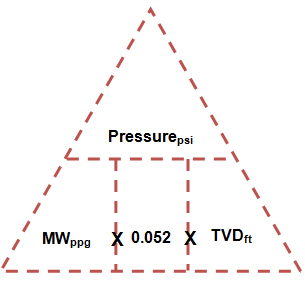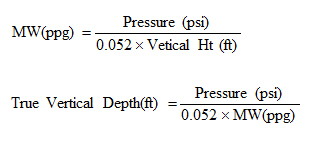# Well Control Formulas Part 1

I would like to share with you about well control formulas that might be advantageous for you in the future. I seperate into several parts for you to easy to follow.Before going into all calculations, I would like to emphasize about rounding rules that we will use for well control calculation.

1. Kill Mud Density: We must round up to one decimal place from a calculated kill mud density. For example, if we have the calculated kill mud density of 13.32 ppg, we will round it up to 13.4 ppg.

2. Leak Off Test (LOT): We must round down to one decimal place from a calculated Leak Off Test value. For example if we have the theoretical LOT of 15.57 ppg, we will round down to 15.5 ppg.

When any of the above values have been calculated, the rounded numbers must be used in subsequent calculations.

## Well Control Course Equations Part 1

Pressure (P) – psi

Pressure (psi) = Force (lb) / Area in Square Inch (in 2)

Mud Gradient ( Psi/ft ) = Mud weight ( PPG) x 0.052

Hydrostatic Pressure (HP) – psi

Hydrostatic Pressure (HP) = Mud weight (PPG) x 0.052 x TVD (ft)

You may have seen this triangle before but I need to emphasize on this because it will let you see all relationship of the hydrostatic pressure.If you want to solve for MW or TVD, fill in the known information in these equations below;Bottom Hole Pressure (BHP) – psi

Under static condition

Bottom Hole Pressure (BHP) = Hydrostatic Pressure (HP) + Surface Pressure (SP)

Under dynamic condition, use the following equation.

Bottom Hole Pressure (BHP) = Hydrostatic Pressure (HP) + Surface Pressure (SP) + Frictional Pressure (FrP)

Note: Frictional pressure acts opposite way of flow direction.

Formation Pressure (FP) – psi

After get Shut In Drill Pipe Pressure, you can calculate the formation pressure from this following relationship;

Formation Pressure (FP) = Hydrostatic Pressure (HP) + Shut-In Drill Pipe Pressure (SIDPP)Formulas and Calculations for Drilling, Production and Workover, Second Edition

Share the joy1.Prabin Chandra Rabha says: